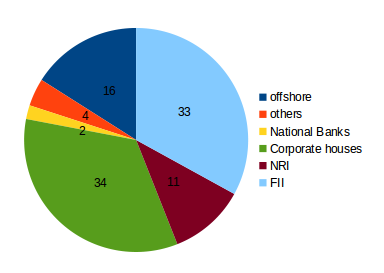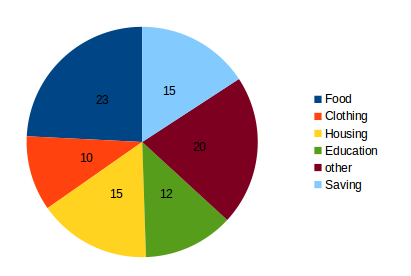## Data Interpretation For SBI PO : Set – 611) In the corporate sector, approximately how many degrees should be there in the central angle ?

a) 120

b) 121

c) 122

d) 123

e) None of these

c)

34*3.6=122.4

2) If the investment by NRI’s are Rs 4,000 crore, then the investments by corporate houses and FII’s together is:

a) 24,000 crore

b) 24,363 crore

c) 25,423 crore

d) 25,643 crore

e) None of these

b)

(67/11)*4000= 24363.6364

3) What percentage of the total investment is coming from FII’s and NRI’s ?

a) 33 %

b) 11 %

c) 44 %

d) 22 %

e) None of these

c)

(33+11)=44

4) If the total investment other than by FII and corporate houses is Rs 335,000 crore, then the investment by NRI’s and Offshore funds will be (approximately) ?

a) 274,100

b) 285,600

c) 293,000

d) Cannot be determined

e) None of these

a)

Investment other than NRI and corporate houses is 33% = 335000. Also, investment by offshore funds and NRI’s is equal to 27%.

Hence, 27 x 335,000/33 = 274 090.909

5) If the total investment flows from FII’s were to be doubled in the next year and the investment flows from all other sources had remained constant at their existing levels for this year, then what would be the proportion of FII investment [/su_spoiler]in the total investment into India Bonds next year (in US \$ millions) ?

a) 40%

b) 50%

c) 60%

d) 70%

e) None of these

b)

FII’s currently account for 33 out of 100.If their value is doubled and all other investments are kept constant then their new value would be 66 out of 133 = approximately equal to 50%

The Values are given in (%)6) If the total amount spent during the year 1998 was Rs.46000, the amount spent on food was:

a) Rs.2000

b) Rs.12447

c) Rs.23000

d) Rs.2300

e) None of these

b)

The total amount spent = Rs 46000

This is 85% of the above pie chart.

The value in the pie-chart= Rs 46000/.85 = Rs. 54118

The amount spent on food was =23% of 54118= (23/100)x46000 = Rs. 12447

7) If the total amount spent was Rs.46000, how much was spent on clothing and housing together?

a) Rs.11500

b) Rs.11050

c) Rs.13529

d) Rs.15000

e) None of these

c)

The total amount spent = Rs 46000

This is 85% of the above pie chart.

The value in the pie-chart= Rs 46000/.85 = Rs. 54118

Total share of clothing and housing = 10% + 15% = 25%

The total amount spent on clothing and housing = 25% of 54118 = (25/100) x 46000= Rs. 13529

8) The ratio of the total amount of money spent on housing to that spent on education was:

a) 5: 2

b) 2: 5

c) 4: 5

d) 5: 4

e) None of these

d)

The data is given as a pie chart. So to calculate the ratio of the amount spent, we do not need the actual amount spent as it will be cancelled out in the final calculations.

The amount spent on housing is 15% and on education is 12% of the total.

Hence, the required ratio is 15: 12 = 5: 4.

9) The given pie-chart shows that the maximum amount was spent on:

a) Food

b) Housing

c) Clothing

d) Others

e) None of these

a)

10) If the total expenditure of the family for the year 1998 was Rs.46000, the amount the family saved during the year is:

a) Rs.1500

b) Rs.15000

c) Rs.6900

d) Rs.8117

e) None of these